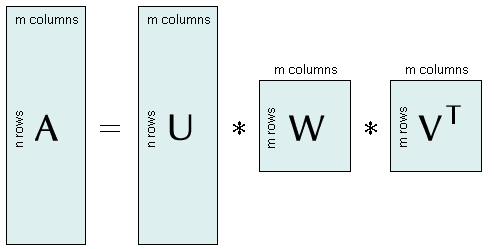The SDL Component Suite is an industry leading collection of components supporting scientific and engineering computing. Please visit the SDL Web site for more information....## SingValDecompUnit: SDL_math2 Class: none Declaration: function SingValDecomp (MatAU, MatV: TMatrix; VecW: TVector): boolean;

When trying to solve linear equation systems which are singular or "near singular" by Gaussian elimination or by LU decomposition these methods fail, or result in unstable solutions. In such cases the solution can be found by a method called singular value decomposition (SVD).

SVD is based on the theorem of linear algebra which states that a matrix A (m columns, n rows) can be transformed into a product of three matrices U, W, and VT which have specific properties: (1) the matrices U and V have orthonormal columns, (2) the matrix V is quadratic, (3) the matrix W is a diagonal matrix with all non-diagonal elements equal to zero.The function SingValDecomp transforms the matrix MatAU into the matrices U, W, and V. The matrix U replaces the data in MatAU ; the diagonal matrix W is stored as a vector (VecW ) which contains the diagonal elements. SingValDecomp returns TRUE if the calculation was successful.

 Hint: The user has to ensure that the dimensions of the matrices MatAU and MatV, and of the vector VecW correspond to each other. Otherwise the results will be wrong.

Last Update: 2016-Oct-02